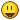# Questions re Plasmac

• Clive S
• Offline
• Platinum Member
•23 Jan 2020 14:34 #155547 by Clive S
Questions re Plasmac was created by Clive S
Q1. Is it possible to reduce the width of the screen by about 15mm. I have to move the screen over the click the max button to fill the screen to the correct size.

Q2. In the ini file re.

[AXIS_Z]
# set to double the value in the corresponding joint
MAX_VELOCITY = 175 Do you mean set this to double
# set to double the value in the corresponding joint
MAX_ACCELERATION = 1500

[JOINT_1]
AXIS = Z
MAX_VELOCITY = 175 or this
MAX_ACCELERATION = 1500

STEPGEN_MAX_VEL = 200 or this
STEPGEN_MAX_ACC = 2500

As a lot of general ini files have this in it
# The values below should be 25% larger than MAX_VELOCITY and MAX_ACCELERATION
STEPGEN_MAXVEL = 250
STEPGEN_MAXACCEL = 1875

So I am a bit confused !

Q3. I am getting an error message on startup. This bit might be better in the general forum

hm2/hm2_7i96.0: stepgen.01.maxvel is too big for current step timings & position-scale clipping to max possible

SCALE = -800
DIRSETUP = 2500
DIRHOLD = 2500
STEPLEN = 3500
STEPSPACE = 3500

23 Jan 2020 15:57 - 23 Jan 2020 15:58 #155555 by PCW
Replied by PCW on topic Questions re Plasmac

hm2/hm2_7i96.0: stepgen.01.maxvel is too big for current step timings & position-scale clipping to max possible

STEPGEN_MAX_VEL = 200
...
SCALE = -800
DIRSETUP = 2500
DIRHOLD = 2500
STEPLEN = 3500
STEPSPACE = 3500

The warning is saying that a maximum velocity of 200 mm/s and a scale of -800
would requite a step rate of 160 KHz (200*800) but the maximum possible step rate
with your step length and step space settings is lower than this ( 1/(3500ns +3500ns) = ~143 KHz)
Last edit: 23 Jan 2020 15:58 by PCW.
The following user(s) said Thank You: Clive S

• Clive S
• Offline
• Platinum Member
•23 Jan 2020 16:29 - 23 Jan 2020 17:15 #155559 by Clive S
Replied by Clive S on topic Questions re Plasmac

The warning is saying that a maximum velocity of 200 mm/s and a scale of -800
would requite a step rate of 160 KHz (200*800) but the maximum possible step rate
with your step length and step space settings is lower than this ( 1/(3500ns +3500ns) = ~143 KHz)

Ah light bulb moment so I need to reduce the step length and spacing 2000ns as a min. ~250KHz This is using a 7i96

Thanks yet again Peteredit wrongly calculated math
Last edit: 23 Jan 2020 17:15 by Clive S.

23 Jan 2020 16:36 #155560 by PCW
Replied by PCW on topic Questions re Plasmac
2000+2000 would get you to 250 KHz Stepgen max velocity
(312.5 mm/sec) and a axis/joint max velocity of 250 mm/sec
(assuming your drives can do that)
The following user(s) said Thank You: Clive S

23 Jan 2020 23:08 - 24 Jan 2020 00:15 #155591 by phillc54
Replied by phillc54 on topic Questions re Plasmac

Q1. Is it possible to reduce the width of the screen by about 15mm. I have to move the screen over the click the max button to fill the screen to the correct size.

If you are using Axis then you could reduce the font size in the ini file.
```# the font for the Axis GUI, if not specified 'sans 10' will be used
# valid font sizes are from 9 to 15
FONT                    = sans 11```

EDIT: I forgot to mention that if you use Axis and want it to start maximised then you can set that in the ini file as well:
```# the window size for the Axis GUI, 0 = minimum size to suit font, 1 = maximized
MAXIMISED               = 1```

Q2. In the ini file re.

[AXIS_Z]
# set to double the value in the corresponding joint
MAX_VELOCITY = 175 Do you mean set this to double
# set to double the value in the corresponding joint
MAX_ACCELERATION = 1500

[JOINT_1]
AXIS = Z
MAX_VELOCITY = 175 or this
MAX_ACCELERATION = 1500

STEPGEN_MAX_VEL = 200 or this
STEPGEN_MAX_ACC = 2500

As a lot of general ini files have this in it
# The values below should be 25% larger than MAX_VELOCITY and MAX_ACCELERATION
STEPGEN_MAXVEL = 250
STEPGEN_MAXACCEL = 1875

So I am a bit confused !

It is referring to the first two with the identical name.

Here are the second two from the docs (note that these are only used if you are using steppers):
```STEPGEN_MAXVEL = 21.0 - Acceleration limit for the step generator. This should be 1% to 10% larger than the joint MAX_ACCELERATION. This value improves the tuning of stepgen’s "position loop". If you have added backlash compensation to an joint then this should be 1.5 to 2 times greater than MAX_ACCELERATION.

STEPGEN_MAXVEL = 1.4 - Older configuration files have a velocity limit for the step generator as well. If specified, it should also be 1% to 10% larger than the joint MAX_VELOCITY. Subsequent testing has shown that use of STEPGEN_MAXVEL does not improve the tuning of stepgen’s position loop.```
So you could safely remove STEPGEN_MAXVEL and the only reason to change STEPGEN_MAXVEL would be if you used the BACKLASH setting.